# The Mathematics of Concurrent Codes

(Last Mod: 27 November 2010 21:37:20 )

NOTE: WORK VERY MUCH IN PROGRESS

## Glossary

The following terminology is used extensively in the discussions regarding concurrent codes.

• message - The data to be transmitted in a single packet, consisting of exactly m bits.
• codeword - The encoded data, consisting of exactly c bits.
• packet - A string of bits one codeword long that may or may not contain codewords superimposed on each other.

## Expected Codeword and Packet Density

The codeword for a message is constructed by placing marks pseudorandomly, according to a hash function, in an initially empty codeword. If N marks are placed in a codeword of length C, then the maximum density that the codeword will have is N/C. However, the expected density will be less than this since some of the N marks will be coincident. Because individual codewords are very sparse, this effect is minimal. However, as codewords are superimposed to form packets approaching the critical density the effect becomes more pronounced.

To determine the expected packet density as a function of the number of marks placed into it, we note that at any given point in the process we have placed k marks which has resulted in n(k) distinct marks in the packet. The probability that the k+1 mark will add a new distinct mark is simply the fraction of spaces remaining in the packet at that time. Hence, the expected number of distinct marks after k+1 marks are added is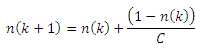.

This can be rewritten as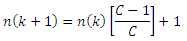.

This recursive relation can be solved in closed form by noting the pattern when n(k+i) is expressed in terms of n(k) and then noting that n(0) is 0 to yield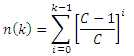.

This series has the well known solution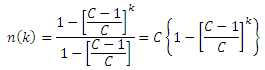.

Since the packet density is obtained by dividing the number of marks in the packet by the length of the packet, we get that the expected mark density of a packet of length C with N marks is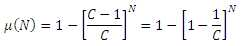.

While this is the exact solution (for the expected number of marks, not the actual number of marks since this is a stochastic system), the numbers involved can result in significant round-off errors if used directly. We can obtain a very good approximation that is easy to compute by noting that, for codewords of practical length, that (1/C)<<1. We can therefore exploit the fact that ln(1+x) is approximately equal to x to obtain.

Where the nominal packet density, μN, is equal to N/C.

A reasonable question to consider is just how good this exponential approximation is. The figure below shows that the match is quite good for codeword lengths as short at 10 and extremely good for lengths in excess of 100. Since practical codeword lengths would seldom be less than 10,000, the exponential equation is a very good model.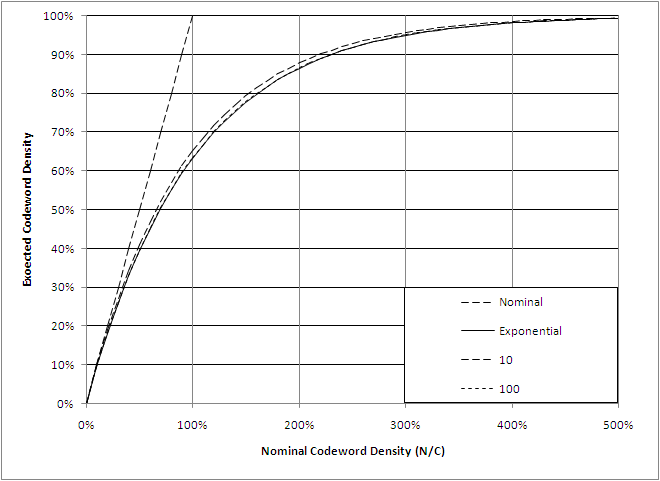On the other end of the scale, a reasonable question is whether simply using the nominal density is good enough. As the figure above shows, the deviation starts to become noticeable above about 20%. By examining this portion of the curve more closely in the figure below, we see that for most purposes the nominal density is an excellent model for densities below a few percent while, provided some thought is given to the accuracy requirements, it is still useful into the 10% to 20%.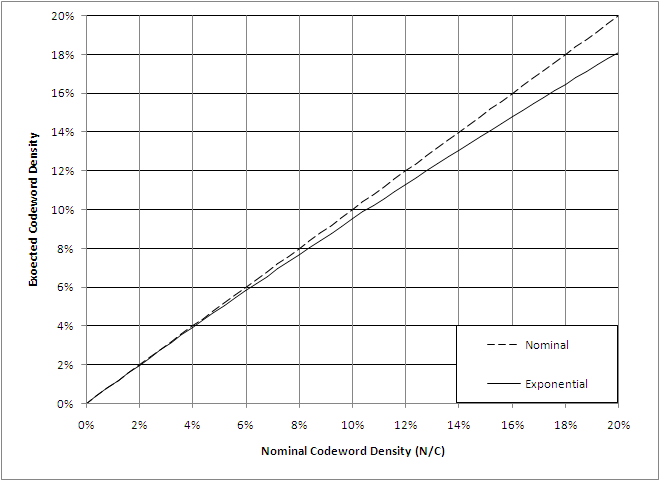The results above are technically only applicable to single codewords having the given nominal density. When multiple codewords are superimposed, the expected density falls further because the BBC encoding means that the first bits in the messages being encoded do not each add fully independent marks to the packet - a message adds independent marks only after it no longer shares a prefix with any other messages already in the packet. For instance, consider the case in which 256 codewords are superimposed. If all of the marks in all of the codewords were independent, then the first eight bits of all of the messages would add 2048 independent marks to the packet. However, because of the BBC encoding algorithm, they add, at most, 510 such marks, and this only if every possible 8-bit prefix is used.

If the length of the messages being encoded is significantly greater than the base-2 log of the number of messages, then this effect is relatively minor. For instance, if the messages are 100 bits in length and 256 messages, each with a unique 8-bit prefix, are superimposed, then the number of independent marks is 24,064 instead of 25,600, or 94% of the fully independent case. However, the other extreme can't be written off so easily. For instance, consider 256 messages in which the messages differ only in the last eight bit positions. In this case the first 92 bits of all of the messages would encode identically and would thus add only 92 marks to the packet. The remaining eight bits, and the 510 independent marks they contribute, would result in only 602 independent marks which is less than 2.5% of the fully independent number. The actual number of independent marks in the packet lies somewhere in the vast gulf between these two bounds.

We can find the estimated number of independent marks in a packet given M messages if we assume that all M messages are independently chosen. At each level, k, of the binary tree representing the possible messages, there are 2k nodes. For randomly selected messages, each node will be chosen with equal probability. Thus, on average, the number of nodes that are passed through obey the same statistics as the number of distinct marks in a codeword of length 2k having M independent marks placed into it.

For M independent messages of length L, the number of independent marks is given by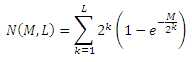.

For k < log2(M), this approaches 2k very rapidly, while for k > log2(M), it approaches M very rapidly, as shown in the figure below (for M=256).If the curve were truly symmetric about the center point, then the expected number of independent marks superimposed in a packet would be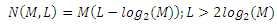.

If these marks are placed in a packet of length C, then the expected packet density would be.

For the example we have been working with, where were have 256 messages that are each 100 bits in length, the approximation above estimates 23,552 independent marks, which is 94% of the case in which all of the marks are independent. If these are then encoded into a message packet that is 1000 times the length of a message (i.e., the codeword length is 100,000 bits), then the expected codeword density would be 21.0%. Direct computation of the expected number of independent marks yields 23,834 and an expected density of  21.2%.

## Critical Mark Density

The critical mark density is the packet mark density above which, statistically, the decoder workload increase exponentially. From a practical standpoint, a decoder operating near the critical density will become bogged down while exploring the decoding tree and will likely have to be terminated prior to being able to completely decode the packet.

### Without Interstitial Checksum Bits

For codecs not configured to use interstitial checksum bits, the critical packet density is 50%. Below that, the number of potential messages having a prefix of length k that are covered by the packet rises exponentially at first, but then quickly stabilizes at a steady state value after which hallucinations are terminated, on average, at the same rate they are spawned. Once the terminal checksum bits are reached, hallucinations that have survived to that point are exterminated exponentially leaving few, if any to be passed up to the higher layers.

The workload of the decoder is mostly proportional to the number of nodes in the potential message tree that must be visited, thus a very useful proxy for the workload is the number of unique message prefixes, P(k), exist after the kth stage of decoding. Consider a packet of density μ containing M legitimate messages. Keep in mind that illegitimate senders are capable of transmitting legitimate messages; while higher layers of the stack may be able to discriminate against them based on embedded authentication information, the decoder is fundamentally incapable to doing so and, hence, sees any properly encoded block of bits as a legitimate message. After partially decoding the packet through bit k (of the padded message), there will be P(k) branches that must be explored further; some of these will be due to the legitimate messages while the remainder will be due to hallucinations that have survived to that point.

Assuming all of the messages to be independent and randomly distributed throughout the message space -- an assumption that must be revisited in the case of intentional attacks -- the number of branches due to legitimate messages will quickly rise to M, the number of legitimate messages, and will remain there for the remainder of the decoding process. This is expected to happen by about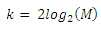.

Given the workload, P(k), at the kth stage of decoding, the expected workload at the (k+1)th stage can be found by noting, for each prefix remaining at this state, two prefixes must be explored and potentially pruned to determine .P(k+1). Assuming that we are far enough into the decoding process such that each legitimate message has a unique prefix, then for each prefix of a legitimate message, the branch containing the legitimate message will survive while the other branches may survive and spawn a new hallucination with a probability that is equal to the the packet density, μ. For each prefix that is not the prefix of a legitimate message, each of the possible branches may spawn a new hallucination with this same probability.

Thus the following relationships apply: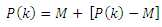,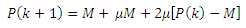.

In steady state, the number of branches at each decoding stage is stable and, therefore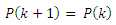.

Several observations can be made at this point:

1. At a packet density of μ=0.5, PSS is infinite. This is the critical mark density for a codec that is not using interstitial checksum bits.
2. For a packet density of μ=1/3, the PSS=2M, meaning that for each legitimate message there is one hallucination.
3. The packet density at which PSS=10M is μ=47+%, meaning that the decoder can operate very close to the critical density while remaining well behaved.

Once the last bit from the actual message has been decoded all that remains is to decode the terminal checksum bits. Since it is known a priori that these have a value of zero, only that branch is examined. As a result, no new hallucinations can be spawned and existing hallucinations are exterminated exponentially since, on average, only μ of them survive each decoding additional stage. Since the expected number of hallucinations at the beginning of the checksum bits is

Terminal Hallucinations = M(μ)/(1-2μ)

the number of hallucinations expected to survive K terminal checksum bits is

Realized Hallucinations = M(μ(K+1))/(1-2μ)

Thus, the number of hallucinations that survivesteady state P(k) one of these will survive (, .the following:

To put some numbers to these equations, let's assume that 100 messages are superimposed and that the packet density is 33%. In order to have a probability of less than 1% than any hallucinations survive the decoding process only nine terminal checksum bits are necessary. To take matters to a bit of an extreme, consider 1000 messages superimposed such that the packet density is driven to 49.97%, which is the required level to generate 1000 hallucinations per legitimate message. With just 24 checksum bits, the odds of a single one of the million expected terminal hallucinations becoming realized is less 6%, while using 32 checksum bits reduces this to than 0.025%.

### With Interstitial Checksum Bits

The development thus far has assumed that interstitial checksum bits have not been used. When employed, they permit the recovery of messages from packets with arbitrarily high mark densities. While it remains true that the number of message prefixes in the decoder will still grow exponentially fast when the packet density rises above 50% (assuming each bit places one mark in the codeword), each group of interstitial checksum bits exterminates these hallucinations exponentially, as well. Thus, in steady state, the expected number of prefixes in the decoder will oscillate between a high limit, reached just prior to decoding a group of interstitial checksum bits, and a low limit, reached just after.

Once in steady state, the expected number of message prefixes in the decoder after a group of S interstitial checksum bits has been decoded is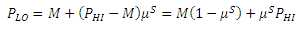.

The expected number of message prefixes just before the next group of S interstitial checksum bits is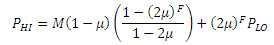.

In steady state, the upper and lower limits are cyclic; solving for the upper limit yields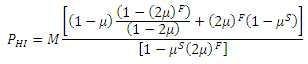.

Although it appears that the numerator has the potential to become unbounded, the denominator in the first term of the numerator factors into the numerator of that term, since (1-x) is a factor of (1-xN), and hence this is a removable singularity. Thus, the critical density is determined by the denominator becoming singular, which occurs at a density of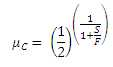.

Notice that if there are no interstitial checksum bits (i.e., S=0), then the expected result of μC=0.5 is obtained. In the case of alternating checksum bits (i.e., S=F=1), the critical density is raised to 70.7%. It must be noted that the codeword density is essentially doubled by doing so and the resulting self-jamming reduces the realized processing gain. However, it turns out that, for packet densities above about 25%, the actual decoder workload decreases with this configuration because decoding checksum bits is very efficient and the lower number of hallucinations compensates for the added work.

It is possible to drive the critical density arbitrarily close to unity. For instance, inserting 128 checksum bits between every message bit results in a critical density of  99.5%. This has been demonstrated in simulation and, somewhat remarkably, the decoding workload remains quite tame.

## Multi-Mark BBC

While the combination of a suitable modulation and detection scheme coupled with the proper choice of a detection threshold can result in low mark error rates while keeping space error rates acceptable, the mark error rate will never be identically zero. With Vanilla BBC, any mark error (that is significant) will result in all messages sharing the affected prefix being lost. For simplicity, we will assume that all legitimate messages are represented by independent marks, which is the case as long as only a single codeword is present in any given transmission packet, even if packets are overlapped to decrease transmission latency at the cost of increased overall packet density.

For illustration purposes, let's consider a 256 bit message with an expansion factor of 1000 using Vanilla BBC with 32 terminal checksum bits and using bookend marks in the codeword template. Each codeword thus has a maximum of 290 marks (and most will be very close to this). The loss of even a single mark will result in the loss of all 256 bits of the message. In order to keep the effective bit error rate (BER) below 1%, the mark error rate would have to be on the order of 1 mark error in 29,000 marks, or 34ppm. If the desired BER were 10ppm, then the mark error rate would have to be below 34ppb.

Now consider an encoding scheme in which each mark is replaced by a composite of B marks but, when decoding, only Q of them need to be found in order for the composite mark to be declared present. In other words, we can tolerate Z=(B-Q) missed marks within each composite mark. If the mark error probability is x, then the composite mark error probability is given by that the composite mark will missed is For instance, consider a Q/B configuration of 2/3. With a mark error rate of x, the probability that a legitimate mark would not be found is approximately 3x2. Thus, in order to achieve an overall BER of 10ppm, the mark error rate only needs to be 100ppm instead of 34bbp. To hold the BER to 1%, a mark error rate as high as 0.3% could be tolerated. With a configuration of 3/5